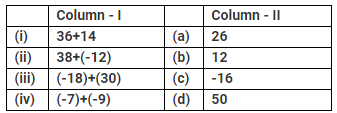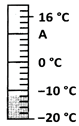Courses

# Test: Integers - 3

## 20 Questions MCQ Test Mathematics (Maths) Class 6 | Test: Integers - 3

Description
This mock test of Test: Integers - 3 for Class 6 helps you for every Class 6 entrance exam. This contains 20 Multiple Choice Questions for Class 6 Test: Integers - 3 (mcq) to study with solutions a complete question bank. The solved questions answers in this Test: Integers - 3 quiz give you a good mix of easy questions and tough questions. Class 6 students definitely take this Test: Integers - 3 exercise for a better result in the exam. You can find other Test: Integers - 3 extra questions, long questions & short questions for Class 6 on EduRev as well by searching above.
QUESTION: 1

### What is the set of negative numbers and whole numbers called?

Solution:

The set of integers comprises negative numbers and whole numbers.

QUESTION: 2

### Which of the following is the greatest negative integer?

Solution:

The greatest negative integer is - 1.

QUESTION: 3

### Which number is neither positive nor negative?

Solution:

Zero has no sign (+ or -). Si it is neither positive nor negative.

QUESTION: 4

What is the number of negative integers between -11 and 11?

Solution:

Excluding -11 and 11, the negative integers between them are -10,-9,-8,......-1.

QUESTION: 5

The opposite of -36 is 36. Which is the only integer that does not have an opposite?

Solution:
QUESTION: 6

Which of these is the smallest positive integer?

Solution:
QUESTION: 7

To which side of 0 on a horizontal number line are the negative integers located?

Solution:
QUESTION: 8

How is the opposite of rise in temperature by 5C denoted?

Solution:
QUESTION: 9

What is the integer representation of walking 3km south?

Solution:
QUESTION: 10

Which of the following integers is greater than –1 and lesser than 7?

Solution:
QUESTION: 11

On a vertical number line, where are the positive numbers placed?

Solution:

Positive numbers are located above 0 and negative numbers are located below 0 on a vertical number line.

QUESTION: 12

What is the opposite of a profit of Rs. 250?

Solution:
QUESTION: 13

Match the following:Solution:
QUESTION: 14

Direction: The figure shows a thermometer that records a winter day's temperature at 5:30 a.m.What is the missing digit on the thermometer denoted by A?

Solution:
QUESTION: 15

The figure shows a thermometer that records a winter day's temperature at 5:30 a.m.It the temperature warms up by 7C, what temperature will it be?

Solution:
QUESTION: 16

The figure shows a thermometer that records a winter day's temperature at 5:30 a.m.If the temperature dropped by 2C, what would it have been?

Solution:
QUESTION: 17

The figure shows a thermometer that records a winter day's temperature at 5:30 a.m.If the temperature starts at-4C, rises by 4C, falls by 3C and then rises again by 6C then what would be the final temperature?

Solution:

(-4C) + (4C) + (-3C) + (6C) =3C

QUESTION: 18

The figure shows a thermometer that records a winter day's temperature at 5:30 a.m.By how much will the temperature have risen if it registers4C?

Solution:

4C-(-12C)=4C+12C =16C

QUESTION: 19

What are the integers between -3 and 3?

Solution:
QUESTION: 20

Between which two integers does -6 lie?

Solution: